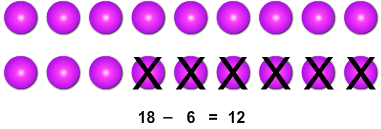# Subtraction Sentences - True/False

Rate 0 stars Common Core
Quiz size:
Message preview:
Someone you know has shared quiz with you:

To play this quiz, click on the link below:

https://www.turtlediary.com/quiz/subtraction-sentences-true-false.html

Hope you have a good experience with this site and recommend to your friends too.

Login to rate activities and track progress.
Login to rate activities and track progress.

If the two sides of a subtraction sentence are equal, then the sentence is said to be true.

For example: Consider the subtraction sentence.

18 – 6 = 12

Let's check if the given subtraction sentence is true.

Find the difference of 18 and 6.18 – 6 equals 12.

So, the given subtraction sentence 18 – 6 = 12 is true.

ds

A B C D E F G H I J K L M N O P Q R S T U V W X Y Z